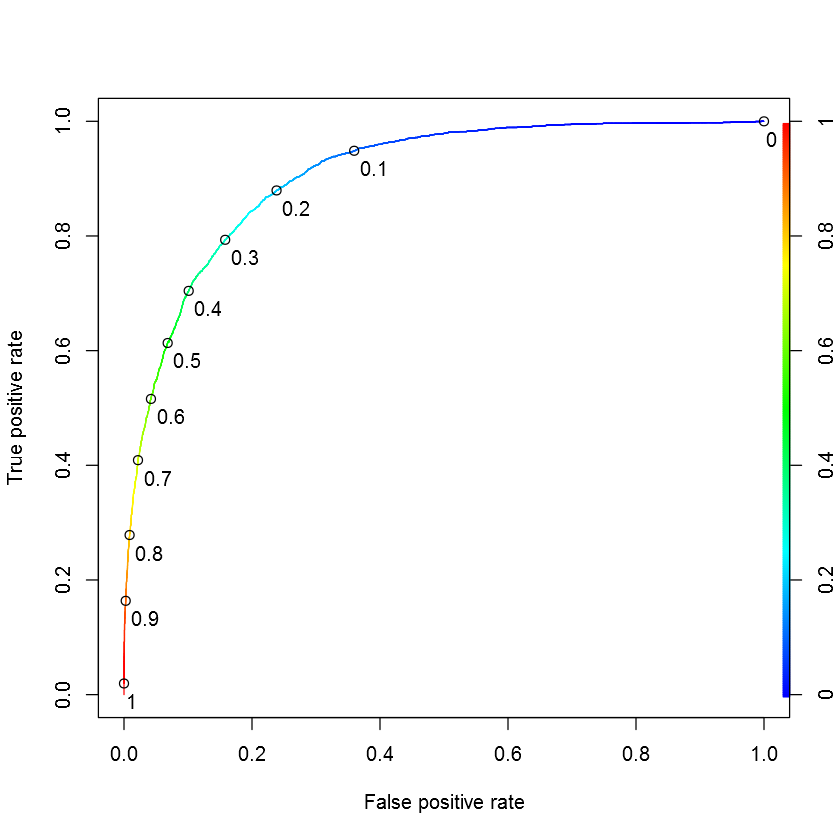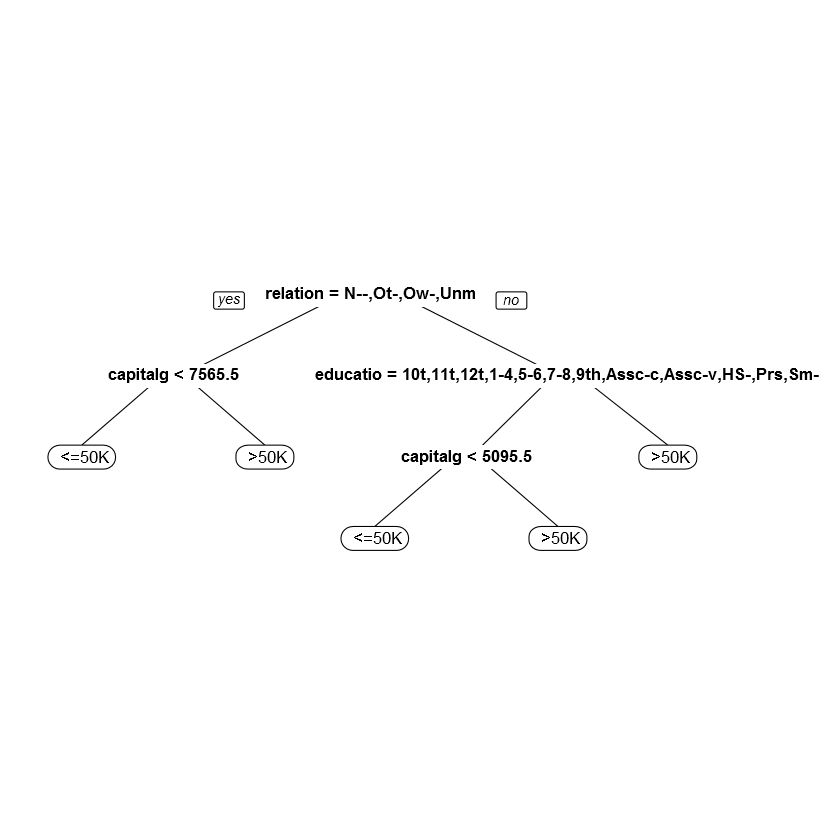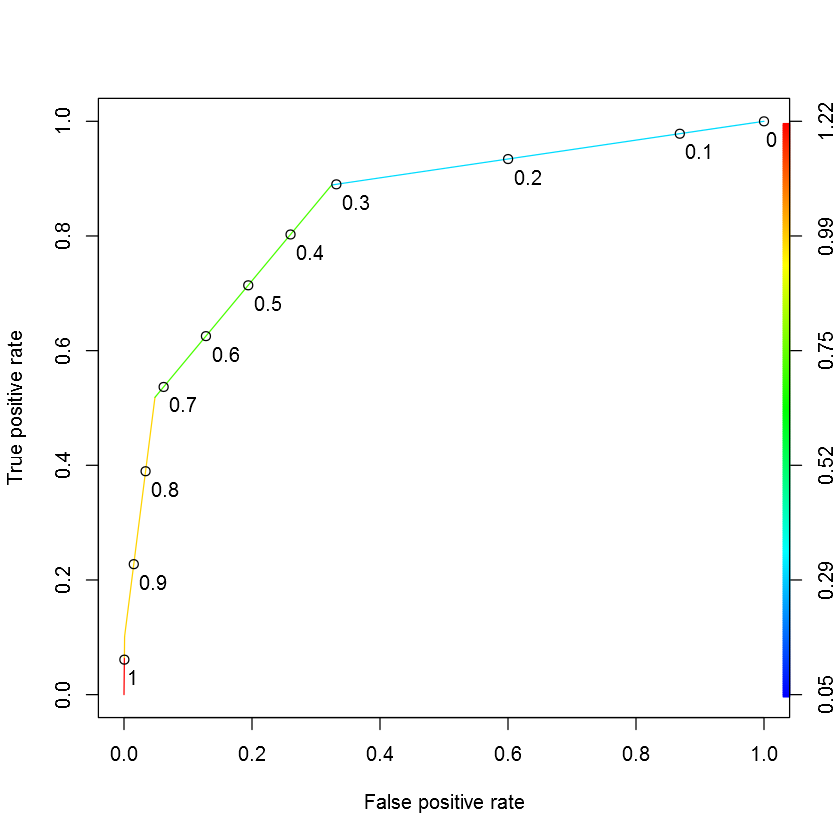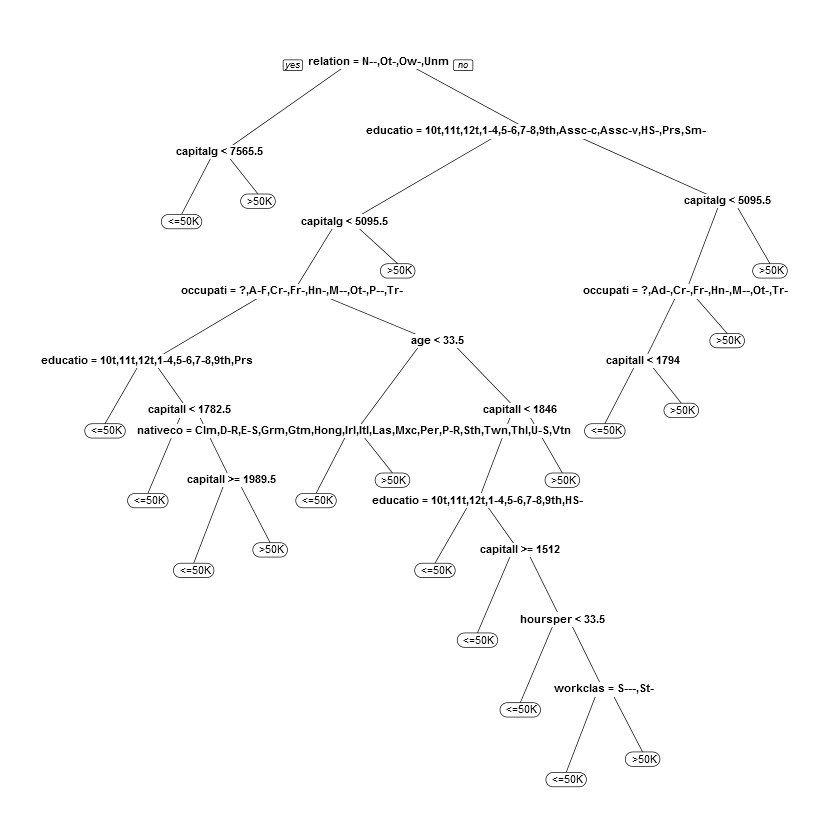# Fisseha Berhane, PhD

#### Data Scientist443-970-2353[email protected] CV Resume### Predicting Earnings from census data¶

The United States government periodically collects demographic information by conducting a census.

In this problem, we are going to use census information about an individual to predict how much a person earns -- in particular, whether the person earns more than $50,000 per year. This data comes from the UCI Machine Learning Repository . The file census.csv contains 1994 census data for 31,978 individuals in the United States. The dataset includes the following 13 variables: age = the age of the individual in years workclass = the classification of the individual's working status (does the person work for the federal government, work for the local government, work without pay, and so on) education = the level of education of the individual (e.g., 5th-6th grade, high school graduate, PhD, so on) maritalstatus = the marital status of the individual occupation = the type of work the individual does (e.g., administrative/clerical work, farming/fishing, sales and so on) relationship = relationship of individual to his/her household race = the individual's race sex = the individual's sex capitalgain = the capital gains of the individual in 1994 (from selling an asset such as a stock or bond for more than the original purchase price) capitalloss = the capital losses of the individual in 1994 (from selling an asset such as a stock or bond for less than the original purchase price) hoursperweek = the number of hours the individual works per week nativecountry = the native country of the individual over50k = whether or not the individual earned more than$50,000 in 1994

##### Install and Load required libraries¶
In :
options(jupyter.plot_mimetypes = 'image/png')

In :
if (!require(caTools)){
install.packages('caTools', repos='http://cran.us.r-project.org')
}

if (!require(ROCR)){
install.packages('ROCR', repos='http://cran.us.r-project.org')
}

if (!require(rpart)){
install.packages('rpart', repos='http://cran.us.r-project.org')
}

if (!require(randomForest)){
install.packages('randomForest', repos='http://cran.us.r-project.org')
}

if (!require(caret)){
install.packages('caret', repos='http://cran.us.r-project.org')
}

if (!require(e1071)){
install.packages('e1071', repos='http://cran.us.r-project.org')
}

if (!require(rpart.plot)){
install.packages('rpart.plot', repos='http://cran.us.r-project.org')
}

if (!require(ggplot2)){
install.packages('ggplot2', repos='http://cran.us.r-project.org')
}


#### Problem 1.1 - A Logistic Regression Model¶

Let's begin by building a logistic regression model to predict whether an individual's earnings are above $50,000 (the variable "over50k") using all of the other variables as independent variables. First, read the dataset census.csv into R. Then, split the data randomly into a training set and a testing set, setting the seed to 2000 before creating the split. Split the data so that the training set contains 60% of the observations, while the testing set contains 40% of the observations. Next, build a logistic regression model to predict the dependent variable "over50k", using all of the other variables in the dataset as independent variables. Use the training set to build the model. Which variables are significant, or have factors that are significant? (Use 0.1 as your significance threshold, so variables with a period or dot in the stars column should be counted too. You might see a warning message here - you can ignore it and proceed. This message is a warning that we might be overfitting our model to the training set.) Select all that apply. In : census<-read.csv("census.csv") set.seed(2000) split<-sample.split(census$over50k,SplitRatio = 0.6)

train<-subset(census, split==TRUE)

test<-subset(census, split==FALSE)
logit1<-glm(over50k~., data=train, family='binomial')
#summary(logit1)  # from this we can see which variables are signiificant

Warning message:
: glm.fit: fitted probabilities numerically 0 or 1 occurred

age, workclass, education, maritalstatus, occupation, relationship, sex, capitalgain, capitalloss, hoursperweek

#### Problem 1.2 - A Logistic Regression Model¶

What is the accuracy of the model on the testing set? Use a threshold of 0.5. (You might see a warning message when you make predictions on the test set - you can safely ignore it.)

In :
prediction<-predict(logit1, type="response",newdata=test)

table<-table(prediction>0.5,test$over50k) accuracy<-sum(diag(table))/(sum(table)) accuracy  Warning message: In predict.lm(object, newdata, se.fit, scale = 1, type = ifelse(type == : prediction from a rank-deficient fit may be misleading Out: 0.855210695019936 #### Problem 1.3 - A Logistic Regression Model¶ What is the baseline accuracy for the testing set? In : table(test$over50k)/(sum(table(test$over50k)))  Out: <=50K: 0.75936205144242 #### Problem 1.4 - A Logistic Regression Model¶ What is the area-under-the-curve (AUC) for this model on the test set? In : # Prediction function ROCRpred = prediction(prediction, test$over50k)

# Performance function
ROCRperf = performance(ROCRpred, "tpr", "fpr")

# Plot ROC curve with colors and threshold labels

as.numeric(performance(ROCRpred, "auc")@y.values)

Out:
0.906159757757142#### Problem 2.1 - A CART Model¶

We have just seen how the logistic regression model for this data achieves a high accuracy. Moreover, the significances of the variables give us a way to gauge which variables are relevant for this prediction task. However, it is not immediately clear which variables are more important than the others, especially due to the large number of factor variables in this problem.

Let us now build a classification tree to predict "over50k". Use the training set to build the model, and all of the other variables as independent variables. Use the default parameters, so don't set a value for minbucket or cp. Remember to specify method="class" as an argument to rpart, since this is a classification problem. After you are done building the model, plot the resulting tree.

How many splits does the tree have in total?

In :
CARTmodel = rpart(over50k ~. , data=train, method="class")

prp(CARTmodel, digits = 6)#### Problem 2.2 - A CART Model¶

Which variable does the tree split on at the first level (the very first split of the tree)?

Ans: relationship

#### Problem 2.3 - A CART Model¶

Which variables does the tree split on at the second level (immediately after the first split of the tree)? Select all that apply.

Ans: education & capitalgain

#### Problem 2.4 - A CART Model¶

What is the accuracy of the model on the testing set? Use a threshold of 0.5. (You can either add the argument type="class", or generate probabilities and use a threshold of 0.5 like in logistic regression.)

In :
prediction<-predict(CARTmodel, type="class",newdata=test)

table<-table(prediction,test$over50k) accuracy<-sum(diag(table))/(sum(table)) accuracy  Out: 0.847392697990775 This highlights a very regular phenomenon when comparing CART and logistic regression. CART often performs a little worse than logistic regression in out-of-sample accuracy. However, as is the case here, the CART model is often much simpler to describe and understand. #### Problem 2.5 - A CART Model¶ Let us now consider the ROC curve and AUC for the CART model on the test set. You will need to get predicted probabilities for the observations in the test set to build the ROC curve and compute the AUC. Remember that you can do this by removing the type="class" argument when making predictions, and taking the second column of the resulting object. Plot the ROC curve for the CART model you have estimated. Observe that compared to the logistic regression ROC curve, the CART ROC curve is less smooth than the logistic regression ROC curve. Which of the following explanations for this behavior is most correct? (HINT: Think about what the ROC curve is plotting and what changing the threshold does.) A) The number of variables that the logistic regression model is based on is larger than the number of variables used by the CART model, so the ROC curve for the logistic regression model will be smoother. B) CART models require a higher number of observations in the testing set to produce a smoother/more continuous ROC curve; there is simply not enough data. C) The probabilities from the CART model take only a handful of values (five, one for each end bucket/leaf of the tree); the changes in the ROC curve correspond to setting the threshold to one of those values. D) The CART model uses fewer continuous variables than the logistic regression model (capitalgain for CART versus age, capitalgain, capitallosses, hoursperweek), which is why the CART ROC curve is less smooth than the logistic regression one. Ans: C. See the figure below #### Problem 2.6 - A CART Model¶ What is the AUC of the CART model on the test set? In : prediction<-predict(CARTmodel,newdata=test)[ , 2] # Prediction function ROCRpred = prediction(prediction, test$over50k)

# Performance function
ROCRperf = performance(ROCRpred, "tpr", "fpr")

as.numeric(performance(ROCRpred, "auc")@y.values)

Out:
0.847025569517672#### Problem 3.1 - A Random Forest Model¶

Before building a random forest model, we'll down-sample our training set. While some modern personal computers can build a random forest model on the entire training set, others might run out of memory when trying to train the model since random forests is much more computationally intensive than CART or Logistic Regression. For this reason, before continuing we will define a new training set to be used when building our random forest model, that contains 2000 randomly selected obervations from the original training set. Do this by running the following commands in your R console (assuming your training set is called "train"):

set.seed(1)

trainSmall = train[sample(nrow(train), 2000), ]

Let us now build a random forest model to predict "over50k", using the dataset "trainSmall" as the data used to build the model. Set the seed to 1 again right before building the model, and use all of the other variables in the dataset as independent variables. (If you get an error that random forest "can not handle categorical predictors with more than 32 categories", re-build the model without the nativecountry variable as one of the independent variables.)

Then, make predictions using this model on the entire test set. What is the accuracy of the model on the test set, using a threshold of 0.5? (Remember that you don't need a "type" argument when making predictions with a random forest model if you want to use a threshold of 0.5. Also, note that your accuracy might be different from the one reported here, since random forest models can still differ depending on your operating system, even when the random seed is set. )

In :
set.seed(1)

trainSmall = train[sample(nrow(train), 2000), ]

rfb = randomForest(over50k~. -nativecountry, data=trainSmall)

prediction<-predict(rfb,newdata=test)



0.002

#### Problem 4.2 - Selecting cp by Cross-Validation¶

Fit a CART model to the training data using this value of cp. What is the prediction accuracy on the test set?

In :
CARTmodel = rpart(over50k ~. , data=train, cp=0.002)

prp(CARTmodel, digits = 6)

prediction<-predict(CARTmodel, type="class",newdata=test)

table<-table(prediction,test\$over50k)
accuracy<-sum(diag(table))/(sum(table))
accuracy

Out:
0.86123055273239#### Problem 4.3 - Selecting cp by Cross-Validation¶

Compared to the original accuracy using the default value of cp, this new CART model is an improvement, and so we should clearly favor this new model over the old one -- or should we? Plot the CART tree for this model. How many splits are there?

18

This highlights one important tradeoff in building predictive models. By tuning cp, we improved our accuracy by over 1%, but our tree became significantly more complicated. In some applications, such an improvement in accuracy would be worth the loss in interpretability. In others, we may prefer a less accurate model that is simpler to understand and describe over a more accurate -- but more complicated -- model.